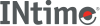﻿ ecvtINtime SDK Help
ecvt

Converts a value to a character string.

```#include <stdlib.h>

char *ecvt (double value, int count, int *dec, int *sign);
```

#### Parameters

`value`
Value to convert.
`count`
Number of digits stored as a string. The function appends a null character \0.
`dec`
Points to an integer value giving the position of the decimal point with respect to the beginning of the string. A 0 or negative integer value indicates that the decimal point lies to the left of the first digit.
`sign`
Points to an integer indicating the sign of the converted number:
 0 Positive. Not 0 Negative.

#### Remarks

Only digits are stored in the string. If the number of digits in `value` exceeds `count`, the low-order digit is rounded. If there are fewer than count digits, the string is padded with 0s.

Obtain the position of the decimal point and the sign of `value` from `dec` and `sign` after the call.

This function uses a single statically allocated buffer for the conversion. Subsequent calls overwrite the result.

#### Return Values

A pointer to the string of digits.

#### Requirements

Versions Defined in Include Link to
INtime 3.0 intime/rt/include/stdlib.h (or)
intime/rt/include/math.h
stdlib.h (or)
math.h
clib.lib